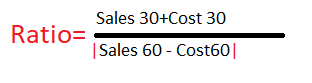# New to Qlik Sense

Discussion board where members can get started with Qlik Sense.

Contributor

## Expression help

Hi Experts,

Can any one please help me to put below formula in Qlik

how to convert denominator mod || in Qlik expression.Here

Sales 30 = Fabs(Sum([Sales 30]))

Sales 60 = Fabs(Sum([Sales 60]))

Cost 30 = Fabs(Sum([Cost 30]))

Cost 60= Fabs(Sum([Cost 60]))

1 Solution

Accepted SolutionsPartner

## Re: Expression help

Did u try?

(

Fabs(Sum([Sales 30]))+Fabs(Sum([Cost 30]))

)

/

Fabs(

Fabs(Sum([Sales 60]))

-

Fabs(Sum([Cost 60]))

)

4 RepliesPartner

## Re: Expression help

Did u try?

(

Fabs(Sum([Sales 30]))+Fabs(Sum([Cost 30]))

)

/

Fabs(

Fabs(Sum([Sales 60]))

-

Fabs(Sum([Cost 60]))

)

Contributor

## Re: Expression help

Hi Mahitha:

Please check the attached file and let know if anything else is your requirement.

-- Shafi

Contributor